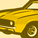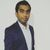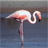# QlikView App Dev

Discussion Board for collaboration related to QlikView App Development.

Announcements
Read about the latest Qlik Community enhancements on the Community News blog!
cancel
Showing results for
Did you mean:Contributor

## need quick help with set analysis

Hi Guys,

I need help in applying set analysis.

I have to do calculation of percentage. I have already applied a set analysis for denominator. I need to again apply a set analysis for the whole calculation i.e., numerator and denominator both. Kindly help me to resolve this problem.

Below is my formula -

=count(proficy_ptn) / Count({\$<[Motor Group]={TM1},[WAG]={WAG2}>}[Motor Group])+count({\$<[Motor Group]={TM2},[WAG]={WAG2}>}[Motor Group])+Count({\$<[Motor Group]={TM1},[WAG]={WAG1}>}[Motor Group])+count({\$<[Motor Group]={TM2},[WAG]={WAG1}>}[Motor Group])

On top of this calculation i need to apply set analysis = {<[motor_type]={AC},[Motor Group]={TM1,TM2},[WAG]={WAG1,WAG2}>}

Regards,

Praveen Prabhu

Labels (1)
• ### Set Analysis

8 RepliesMaster

Hi

You can store this expression in a variable and then use set analysis.

or May be this can help..

sum({<[motor_type]={AC},[Motor Group]={TM1,TM2},[WAG]={WAG1,WAG2}>}
count(proficy_ptn)
/
Count({\$<[Motor Group]={TM1},[WAG]={WAG2}>}[Motor Group])+count({\$<[Motor Group]={TM2},[WAG]={WAG2}>}[Motor Group])+Count({\$<[Motor Group]={TM1},[WAG]={WAG1}>}[Motor Group])+count({\$<[Motor Group]={TM2},[WAG]={WAG1}>}[Motor Group]))Contributor
Author
Nope, this solution is not working.. Any other solution?Contributor
Author
Says nested aggregation not allowed.Master
My bad, it will not work ..
Try storing the data in variable and then use set analysis...Contributor
Author
What aggregation shall i use after storing it in variable?Contributor III

Try this, a little bit simplified. Not sure if understand your requirement correctly.

besides, those values are not number, so should use single quote.

=count({\$<[Motor Group]={'TM1','TM2'},[WAG]={'WAG1','WAG2'}>}proficy_ptn) /
count({\$<[Motor Group]={'TM1','TM2'},[WAG]={'WAG1','WAG2'}>}[Motor Group])Master

The same expression that you have shared before.MVP

May be this

```=Count({<[motor_type] = {AC}, [Motor Group] = {TM1, TM2}, [WAG] = {WAG1, WAG2}>}proficy_ptn)/
(Count({\$<[motor_type] = {AC}, [Motor Group] = {TM1, TM2}, [WAG] = {WAG1, WAG2}>}[Motor Group])+
Count({\$<[motor_type] = {AC}, [Motor Group] = {TM1, TM2}, [WAG] = {WAG1, WAG2}>}[Motor Group])+
Count({\$<[motor_type] = {AC}, [Motor Group] = {TM1, TM2}, [WAG] = {WAG1, WAG2}>}[Motor Group])+
Count({\$<[motor_type] = {AC}, [Motor Group] = {TM1, TM2}, [WAG] = {WAG1, WAG2}>}[Motor Group]))```

Actually, do you mind putting in words as to what exactly are you trying to do here?Community Browser